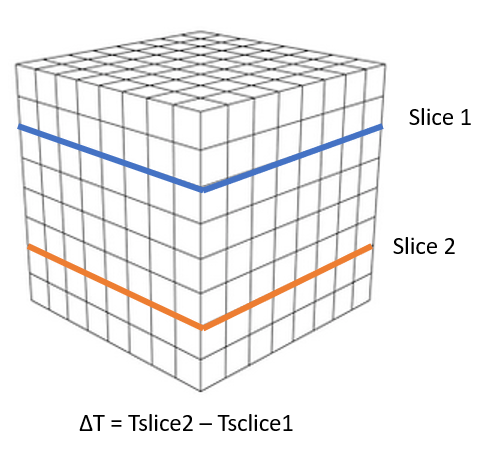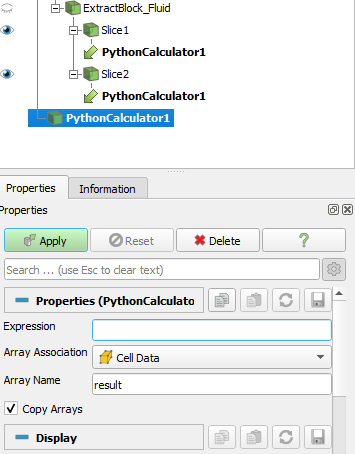# Subtract scalar on different slices

Hello everyone,

I would like to know if, in Paraview, is possible to subtract the temperature of 2 slices that are at different heights in the same computational domain?
The result would be a matrix with the result of the subtraction …

In the attached file you can see an example.If you input is a structured grid, then your two slices should contains the same number of points and cells.

Then computing anything between the two slides would be trivial using PythonCalculator filter.

Something like this? My question now is, how do I refer to the temperature of the slice 1 and slice 2 in the python calculator? Note that the data array name is the same (TEMPERATURE).Thank you.

`inputs.PointData["TEMPERATURE"] - inputs.PointData["TEMPERATURE"]`

Are quotes needed around `TEMPERATURE` ?

2 Likes

edited

Yes,quotes are needed.
And if you are working with cell data use: CellData[“TEMPERATURE”]

1 Like